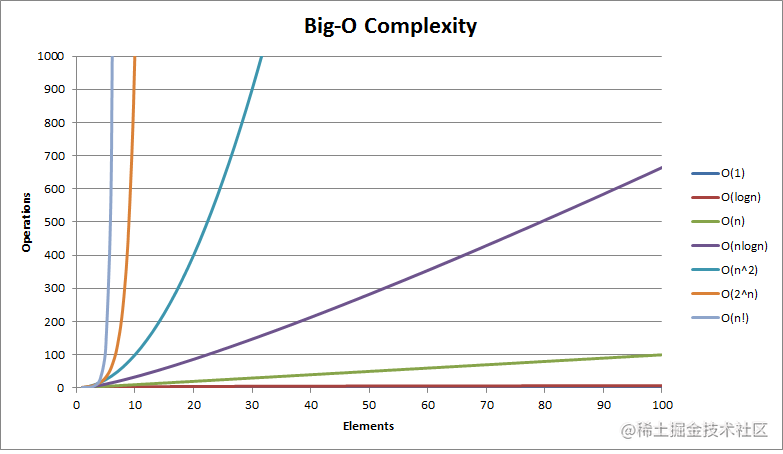# 海绵宝宝带你一起回顾——复杂度分析## 复杂度小结

### 基础概念

• 时间复杂度： 执行当前算法所消耗的时间
• 空间复杂度： 执行当前算法需要占用多少内存空间

### 时间复杂度分析

#### 1. 只关注循环执行次数最多的一段代码

function sum(arr) {
let sum = 0;
for (let i = 0; i < arr.length; i++) {
sum += arr[i];
}
return sum;
}

#### 2. 总复杂度等于量级最大的那段代码的复杂度

function sum(arr) {
let sum = 0;
for (let i = 0; i < arr.length; i++) {
sum += arr[i];
}

let sum1 = 0;
for (let i = 0; i < arr.length; i++) {
for (let j = 0; j < arr.length; j++) {
sum1 = sum1 + arr[i] + arr[j];
}
}

let sum2 = 0;
for (let k = 0; k < 100; k++) {
sum2 += k;
}
return sum + sum1 + sum2;
}

### 常见时间复杂度

• O(1)
• O(logn)
• O(n)
• O(nlogn)
• O(n2)

function sum (arr) {
let i = 1;
let sum = 0;
while(i < arr.length) {
sum += arr[i];
i = i * 2;
}
return sum;
}

function sum (arr) {
let i = 1;
let sum = 0;
while(i < arr.length) {
sum += arr[i];
i = i * 3;
}
return sum;
}### 空间复杂度分析

function print(n)  {
let i = 0;
let a = [];
for (i = 0; i < n; i++) {
a[i] = i * i;
}

for (i = n - 1; i >= 0; i--) {
console.log(a[i])
}
}

### 最好、最坏、平均时间复杂度

function find(arr, x) {
let index = -1;
for (let i = 0; i < arr.length; i++) {
if (arr[i] === x) {
index = i;
}
}
return index;
}

function find(arr, x) {
let index = -1;
for (let i = 0; i < arr.length; i++) {
if (arr[i] === x) {
index = i;
return index;
}
}
return index;
}

$1 * 1/2n + 2 * 1/2n + ...+ n * 1/2n + n * 1/2 = (3n + 1)/4$